Latest Banking jobs   »

# Quantitative Aptitude Quiz For IDBI AM/ Bank of India PO 2023-2nd March

Directions (1 – 10): In each of these questions, two equations numbered I and II are given. You have to solve both the equation and give answer.
(a) if x < y
(b) if x ≤ y
(c) if x > y
(d) if x ≥ y
(e) if x = y or the relationship cannot be established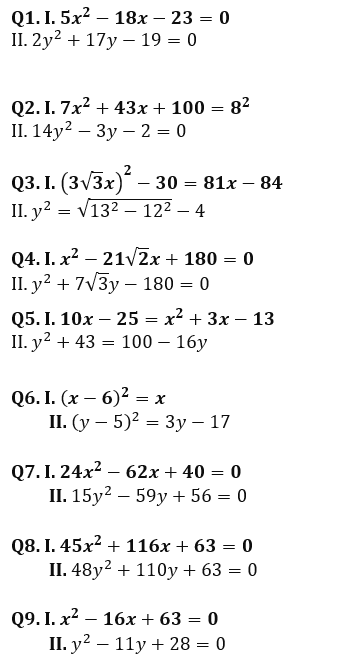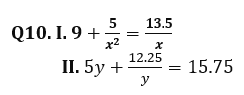Directions (11-15): In the following questions, two equations (I) and (II) are given. You have to solve both the equations and mark the appropriate option.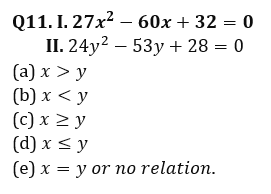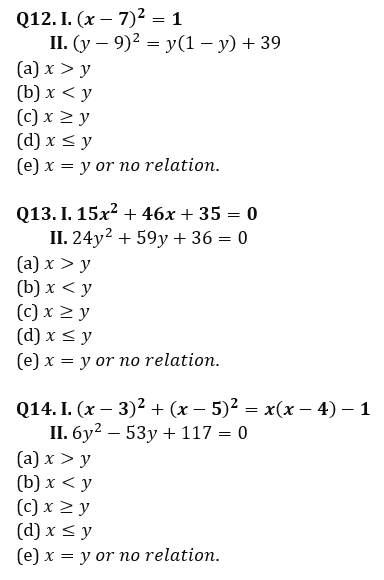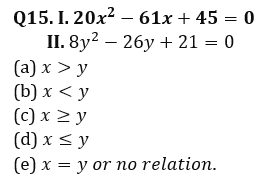Solutions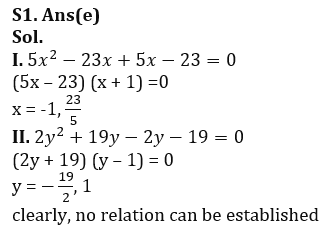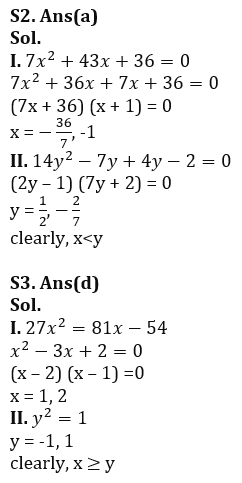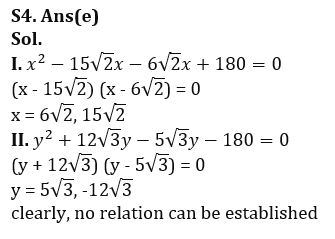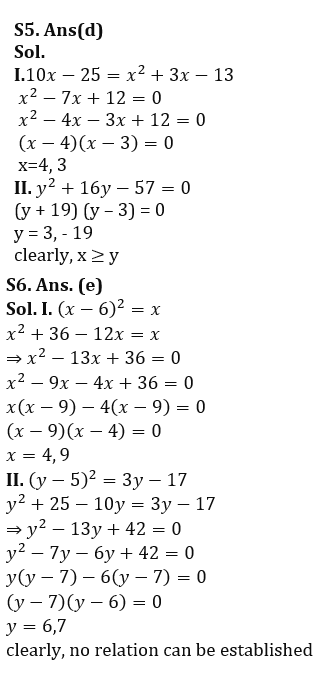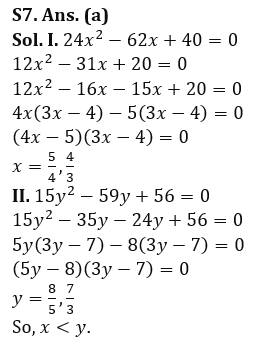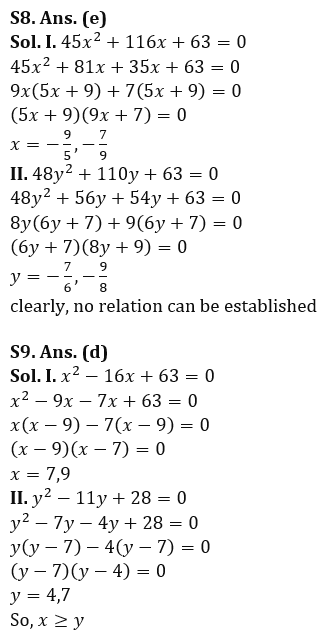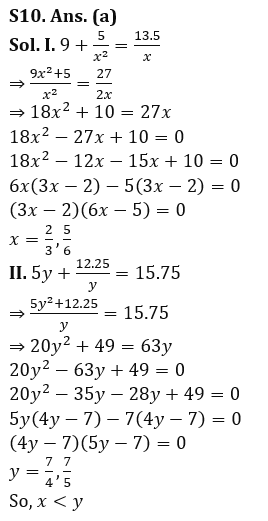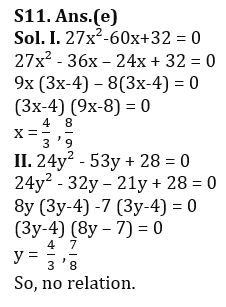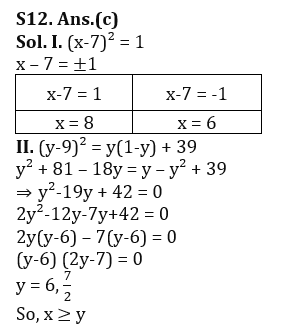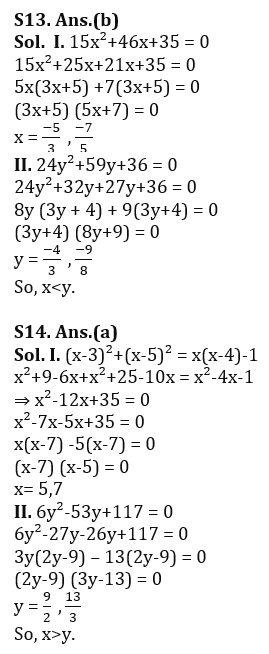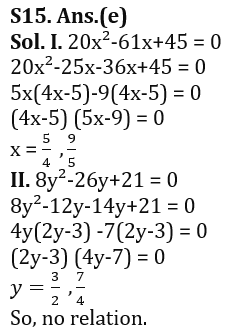.                                                         .                                                                                                                                                                                                                                                                .

## FAQs

### What is the selection process of the IDBI AM?

The selection process of IDBI AM is Online exam and Interview.

#### Congratulations!Union Budget 2023-24: Free PDF